# Excel Financial Functions Part I: NPV, XNPV and PV FunctionsIntroductory financial accounting and finance courses usually suggest that students compute the net present value (NPV) of a sequence of cash flows using cumbersome tables in the textbook’s appendix. There are several things wrong with this approach:

• The tables require the discount rate (expressed on a 1-100) scale to be an integer. What should be done if the discount rate is 5.67%?
• How do you compute NPV when cash flows do not occur periodically, but occur at irregular intervals?
• If I use the tables to compute an NPV for a sequence of cash flows, then I often enter the NPV in Excel. Can’t I just use Excel to compute NPV’s?

Fortunately, Excel has three functions, NPV, XNPV and PV, that can be used to easily compute the NPV of any sequence of cash flows. In this article we will show you how these functions can be used to compute NPV’s.

What is NPV?

Copy this link into your browser to download the Excel file to get started. Figure 1 (see the worksheet “NPV”) shows the sequence of cash flows from two investments. Which investment helps the company more? To answer this question, we need a discount rate that incorporates the time value of money. It is tricky to determine the correct discount rate. (For a discussion of determining the correct discount rate, I recommend Principles of Finance with Excel by Simon Benninga and Tal Mofkadi.)

A dollar today is clearly worth more than a dollar received a year from now. Suppose you can invest \$1 today and turn it into \$1.20 a year from now.

Then \$1 now = \$1.20 a year from now or \$1/1.20 now = \$1 a year from now. This implies that to compute the NPV (value in today’s dollars) of a sequence of annual cash flows, we should multiply the cash flow a year from now by 1/1.2, the cash flow a year from now by 1/1.22, etc. Thus, if the cash flows at Time 0 are received today, and the cash flows at Time 1 and 2 are received one and two years from now, then the NPV of Investment 1 is calculated in Row 7 using the calculation: =-\$10,000  +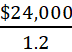+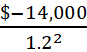=  \$277.

Figure 1: Using the Excel NPV function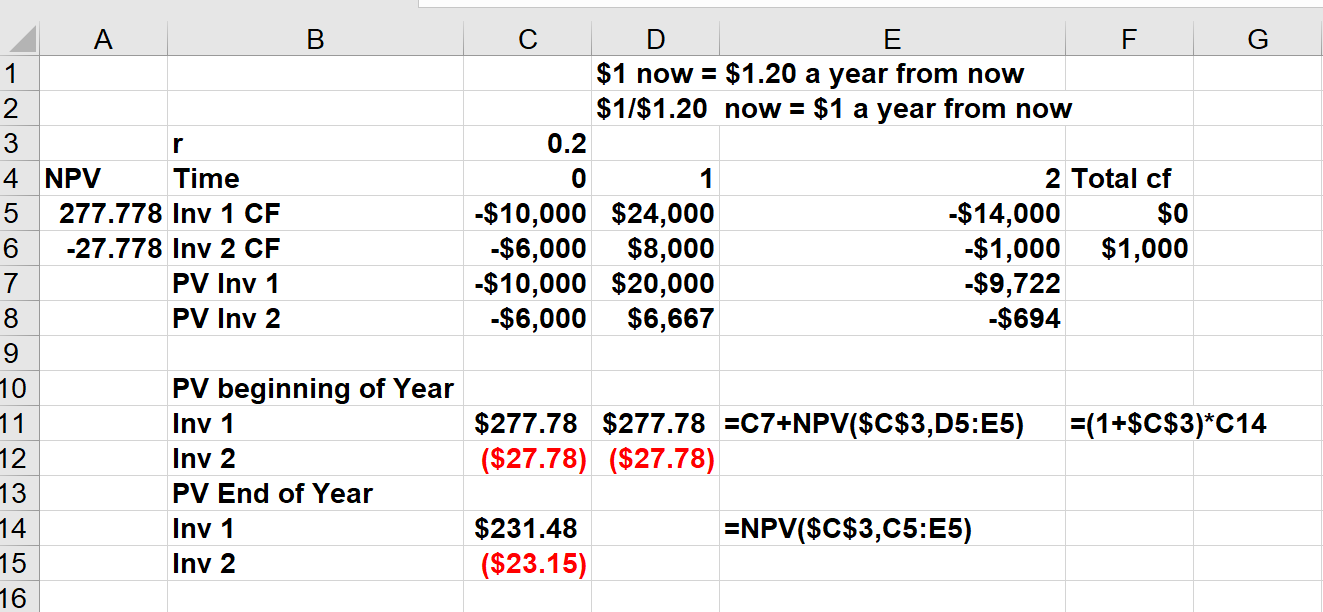Clearly, NPV computations are tedious! Fortunately, the Excel NPV, XNPV, and PV functions come to our rescue.

The NPV function

The Excel NPV function has the syntax NPV(discount rate, range of cash flows.) The function ignores blank cells (put a 0 in if there is no cash flow during a period) and assumes the first cash flow occurs one period from now and cash flows occur at regular intervals. This implies that if cash flows occur at the beginning of a time period, we should separate out the first cash flow and compute the NPV of investment 1 using the formula (cell C11) =C7+NPV(\$C\$3,D5:E5). Copying this formula to cell C12 computes the NPV for Investment 2. Since Investment 1 has a positive NPV and Investment 2 has a negative NPV, we would conclude that Investment 1 helps the company and Investment 2 hurts the company.

If cash flows occur at the end of the year (so cash flow for year 0 occurs at the end of year 0), then copying the formula =NPV(\$C\$3,C5:E5) from C14 to C15 computes the NPV of the two investments.

What about Irregularly Spaced Cash Flows?

Often cash flows don’t occur at regularly spaced intervals. Fortunately, the XNPV function can be used to compute the NPV of a sequence of irregularly spaced cash flows. All you have to is enter the annual discount rate, dates of cash flows and the cash flow values. Then, the XNPV function returns the NPV of the cash flows as of the first listed date. For example, as shown in Figure 2 (and worksheet “XNPV”) the formula  =XNPV(B2,C5:C7,B5:B7) computes the NPV of the cash flows (-\$80.85) as of 1/1/2023.

Figure 2: XNPV function computes NPV as of 1/1/2023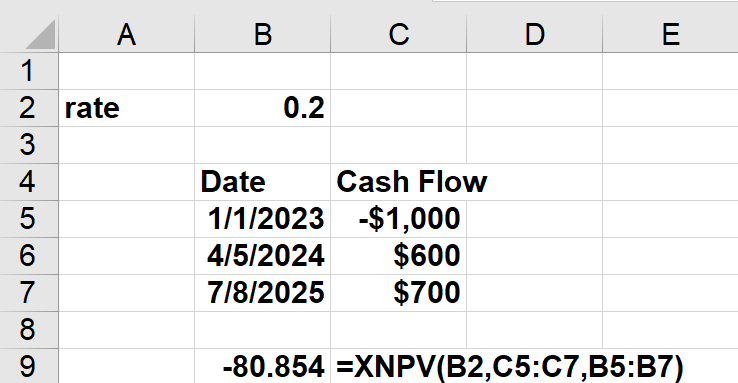Goodbye Annuity Tables!

Often, we want to compute the NPV of an annuity, which is a stream of equal cash flows received at regular intervals. Most introductory accounting and finance books have tables that can be used to compute the present value of an annuity.  Fortunately, Excel’s PV function (see Figure 3 and the worksheet “PV”) can be used to compute the present value of an annuity. The syntax of the PV function is PV(Rate,Nper,Payment,Future_value,Type).

• Rate is the discount rate.
• Nper is number of periods (the discount rate must be consistent with your choice of period length.)
• Payment is annual payment (use a minus sign if you are paying out money and want PV to return a positive value.)
• Future Value (often 0) is the amount of money paid out (minus sign for paid out, positive sign for money received) at the end of the annuity.
• Type is 0 for end of period cash flows and 1 for beginning of period cash flows.

Figure 3: Examples of the PV function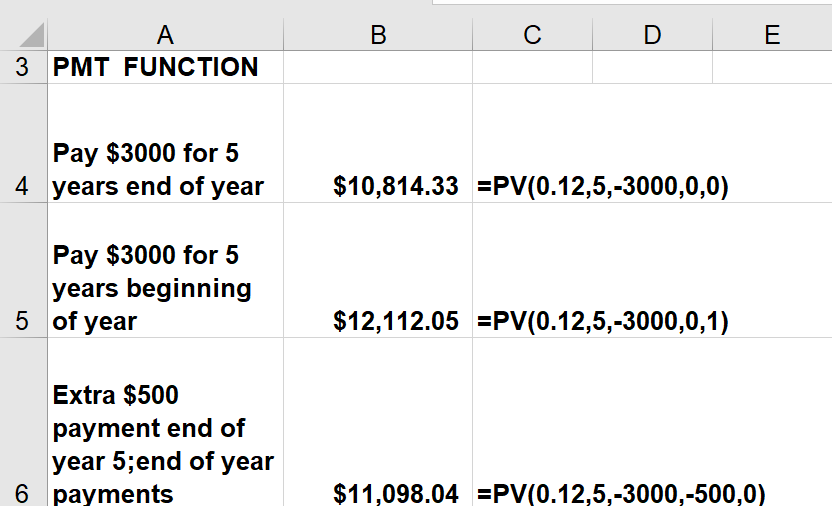Assume that the annual discount rate is 12% and we are going to buy a machine.

• In cell B4, we find that if we pay \$3,000 for five years at the end of each, the present value of our payments is \$10,814.33.
• In cell B5, we find that if the payments are made at the beginning of each year, the present value of the payments is \$12,112.05.
• In cell B6, we find that if we pay for five years \$3000 at the end of each year and make an additional payment of \$500 in five years, then our payments have a present value of \$11,098.04.

Next month, we will discuss the many Excel functions that can be used to analyze loan payments.

### Now Leaving Becker.com

You are leaving the Becker.com website. Once you click “continue,” you will be brought to a third-party website. Please be aware, the privacy policy may differ on the third-party website. Adtalem Global Education is not responsible for the security, contents and accuracy of any information provided on the third-party website. Note that the website may still be a third-party website even the format is similar to the Becker.com website.